Electron. J. Differential Equations, Vol. 2020 (2020), No. 108, pp. 1-20.

### Positive vortex solutions and phase separation for coupled Schrodinger system with singular potential Jin Deng, Aliang Xia, Jianfu Yang

Abstract:
We consider the existence of rotating solitary waves (vortices) for a coupled Schrodinger equations by finding solutions to the singular system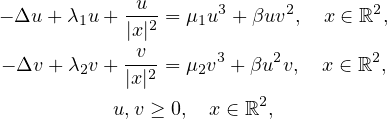where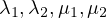are positive parameters,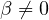. We show that this system has a positive least energy solution for the cases When either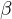is negative oris positive and small or large. Moreover, if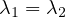, then the solution is unique. We also study the limiting behavior of the least energy solutions in the repulsive case for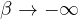, and phase separation.

Submitted March 5, 2020. Published October 30, 2020.
Math Subject Classifications: 35J20, 35B08, 35B40.
Key Words: Schodinger equation; singular potential; Nehari manifold.

Show me the PDF file (386 KB), TEX file for this article.

 Jin Deng School of Mathematics and Statistics Jiangxi Normal University Nanchang, Jiangxi 330022, China email: Dengjin@jxnu.edu.cn Aliang Xia School of Mathematics and Statistics Jiangxi Normal University Nanchang, Jiangxi 330022, China email: aliang_xia@jxnu.edu.cn Jianfu Yang School of Mathematics and Statistics Jiangxi Normal University Nanchang, Jiangxi 330022, China email: jfyang_2000@yahoo.com